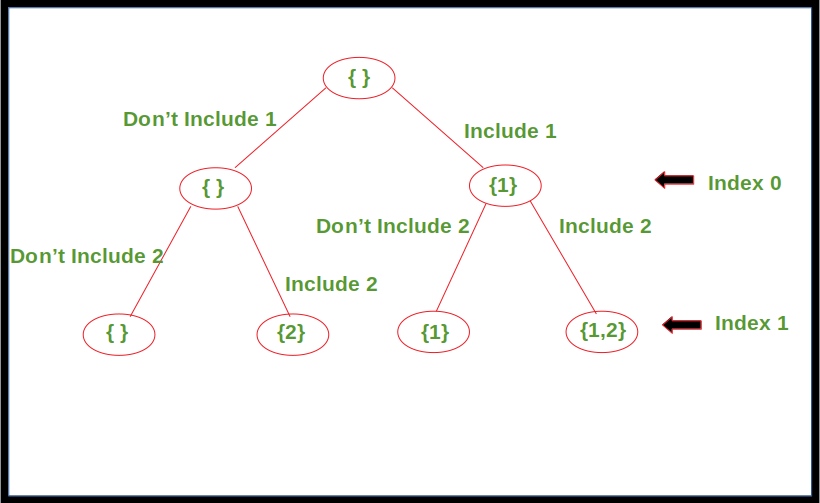# Generating all possible Subsequences using Recursion including the empty one.

• Difficulty Level : Easy
• Last Updated : 25 May, 2022

Given an array. The task is to generate and print all of the possible subsequences of the given array using recursion.

Examples:

```Input : [1, 2, 3]
Output : , , [2, 3], , [1, 3], [1, 2], [1, 2, 3], []

Input : [1, 2]
Output : , , [1, 2], []
```

Approach: For every element in the array, there are two choices, either to include it in the subsequence or not include it. Apply this for every element in the array starting from index 0 until we reach the last index. Print the subsequence once the last index is reached.

Below diagram shows the recursion tree for array, arr[] = {1, 2}.Below is the implementation of the above approach.

## C++

 `// C++ code to print all possible` `// subsequences for given array using` `// recursion` `#include ` `using` `namespace` `std;`       `// Recursive function to print all` `// possible subsequences for given array` `void` `printSubsequences(``int` `arr[], ``int` `index, ` `                       ``vector<``int``> &subarr,``int` `n)` `{` `    ``// Print the subsequence when reach` `    ``// the leaf of recursion tree` `    ``if` `(index == n)` `    ``{` `         ``for` `(``auto` `it:subarr){` `           ``cout << it << ``" "``;` `         `  `         ``}` `      ``if``(subarr.size()==0)` `        ``cout<<``"{}"``;` `      `  `      ``cout< vec;` `     `    `    ``printSubsequences(arr, 0, vec,n);`   `    ``return` `0;` `}`   `// This code is contributed by` `// vivekr4400`

## Java

 `// Java code to print all possible` `// subsequences for given array using` `// recursion` `import` `java.io.*;` `import` `java.util.*;`   `class` `GFG{` `      `  `// Recursive function to print all` `// possible subsequences for given array` `public` `static` `void` `printSubsequences(``int``[] arr, ``int` `index, ` `                                     ``ArrayList path)` `{` `    `  `    ``// Print the subsequence when reach` `    ``// the leaf of recursion tree` `    ``if` `(index == arr.length)` `    ``{` `        `  `        ``// Condition to avoid printing` `        ``// empty subsequence` `        ``if` `(path.size() > ``0``)` `            ``System.out.println(path);` `    ``}` `    `  `    ``else` `    ``{` `        `  `        ``// Subsequence without including` `        ``// the element at current index` `        ``printSubsequences(arr, index + ``1``, path);` `        `  `        ``path.add(arr[index]);` `        `  `        ``// Subsequence including the element` `        ``// at current index` `        ``printSubsequences(arr, index + ``1``, path);` `        `  `        ``// Backtrack to remove the recently ` `        ``// inserted element` `        ``path.remove(path.size() - ``1``);` `    ``}` `    ``return``;` `}`   `// Driver code` `public` `static` `void` `main(String[] args)` `{` `    ``int``[] arr = { ``1``, ``2``, ``3` `};` `      `  `      ``// Auxiliary space to store each path` `      ``ArrayList path = ``new` `ArrayList<>();` `      `  `      ``printSubsequences(arr, ``0``, path);` `}` `}`   `// This code is contributed by Mukul Sharma`

## Python3

 `# Python3 code to print all possible  ` `# subsequences for given array using  ` `# recursion ` `  `  `# Recursive function to print all ` `# possible subsequences for given array ` `def` `printSubsequences(arr, index, subarr): ` `      `  `    ``# Print the subsequence when reach  ` `    ``# the leaf of recursion tree ` `    ``if` `index ``=``=` `len``(arr): ` `          `  `        ``# Condition to avoid printing ` `        ``# empty subsequence ` `        ``if` `len``(subarr) !``=` `0``: ` `            ``print``(subarr) ` `      `  `    ``else``: ` `        ``# Subsequence without including  ` `        ``# the element at current index ` `        ``printSubsequences(arr, index ``+` `1``, subarr) ` `          `  `        ``# Subsequence including the element ` `        ``# at current index ` `        ``printSubsequences(arr, index ``+` `1``,  ` `                            ``subarr``+``[arr[index]]) ` `      `  `    ``return` `          `  `arr ``=` `[``1``, ``2``, ``3``] ` `  `  `printSubsequences(arr, ``0``, []) `   `#This code is contributed by Mayank Tyagi`

## C#

 `// C# code to print all possible` `// subsequences for given array using` `// recursion` `using` `System;` `using` `System.Collections.Generic;` `class` `GFG {` `    `  `    ``// Recursive function to print all` `    ``// possible subsequences for given array` `    ``static` `void` `printSubsequences(``int``[] arr, ``int` `index, List<``int``> path)` `    ``{` `         `  `        ``// Print the subsequence when reach` `        ``// the leaf of recursion tree` `        ``if` `(index == arr.Length)` `        ``{` `             `  `            ``// Condition to avoid printing` `            ``// empty subsequence` `            ``if` `(path.Count > 0)` `            ``{` `                ``Console.Write(``"["``);` `                ``for``(``int` `i = 0; i < path.Count - 1; i++)` `                ``{` `                    ``Console.Write(path[i] + ``", "``);` `                ``}` `                ``Console.WriteLine(path[path.Count - 1] + ``"]"``);` `            ``}` `        ``}` `         `  `        ``else` `        ``{` `             `  `            ``// Subsequence without including` `            ``// the element at current index` `            ``printSubsequences(arr, index + 1, path);` `             `  `            ``path.Add(arr[index]);` `             `  `            ``// Subsequence including the element` `            ``// at current index` `            ``printSubsequences(arr, index + 1, path);` `             `  `            ``// Backtrack to remove the recently` `            ``// inserted element` `            ``path.RemoveAt(path.Count - 1);` `        ``}` `        ``return``;` `    ``}`   `  ``static` `void` `Main() {` `      ``int``[] arr = { 1, 2, 3 };` `       `  `      ``// Auxiliary space to store each path` `      ``List<``int``> path = ``new` `List<``int``>();` `       `  `      ``printSubsequences(arr, 0, path);` `  ``}` `}`   `// This code is contributed by rameshtravel07.`

## Javascript

 ``

output

1 2 3

1 2

1 3

1

2 3

2

3

{}

Time Complexity:Space Complexity:
O(n) , Because of the recursion stack.

My Personal Notes arrow_drop_up
Recommended Articles
Page :